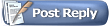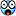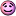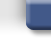Project64 Forums'nother SP problem: with VCR
 FAQ Members List Calendar Search Today's Posts Mark Forums Read#11HatCatAlpha Tester Project Supporter Senior Member Join Date: Feb 2007 Location: In my hat. Posts: 16,236Quote:
 Originally Posted by MarathonManOh, heh. I've only done the VMADs. I haven't done the VMUDs yet. Maybe I mis-spoke somewhere.
I swear that there seems no multiply easier to do than VMUDL.Code:
```void VMUDL(int vd, int vs, int vt, int element)
{
register unsigned int product;
register int i, j;

if (element == 0x0) /* if (element >> 1 == 00) */
{
for (i = 0; i < 8; i++)
{
product = (unsigned short)VR[vs].s[i] * (unsigned short)VR[vt].s[i];
VACC[i].DW = product;
VACC[i].DW >>= 16;
}
}```
[And the need for clamping is impossible here, so sign-clamping can be skipped, even though it is documented to occur.]

So with SSE,
I'm sure you could like, do all multiplications simultaneously (but you must be able to treat all multipliers as unsigned integers ! never signed), then shift all of them to the right by 16 simultaneously (without filling in leading 1's from sign-extension), and boom, you're done.

It's the exact same way with VMADL except you add this to the acc., rather than set the acc. to this result definitively.

I think you can do it no sweat.#12HatCatAlpha Tester Project Supporter Senior Member Join Date: Feb 2007 Location: In my hat. Posts: 16,236Off-topic.

But I h4z a n00b question.

I broke Resident Evil 2 gfx ucode prologue cinema on accident.
I've isolated the cause of the bug to the changes I applied to RSP::VGE.

The bug is not because I made it more accurate to the documentation, but because of some misconception on my part...

it happens if and only if element == 0 and the edge condition where VS == VT ..

Code:
```void VGE(int vd, int vs, int vt, int element)
{
register int i, j;

VCC = 0x0000;
if (element == 0x0) /* if (element >> 1 == 00) */
for (i = 0; i < 8; i++)
if (VR[vs].s[i] > VR[vt].s[i])
{
VCC |= 0x0001 << i;
VACC[i].s[LO] = VR[vs].s[i];
}
else if (VR[vs].s[i] == VR[vt].s[i])
{ /* If vs == vt, either CARRY or NOTEQUAL bit must NOT be set. */
// pass:  VCC |= ((VCO & (0x0101 << i)) != 0x0101 << i) ? 0x0001 << i : 0x0000;
// fail:  VCC |= ~(VCO & (0x0101 << i)) ? 0x0001 << i : 0x0000;
VACC[i].s[LO] = VCC & (0x0001 << i) ? VR[vs].s[i] : VR[vt].s[i];
}
else
{
/* VCC &= ~(0x0001 << i); */
VACC[i].s[LO] = VR[vt].s[i];
}
/* rest of file in case element is not zero */
/* includes write-back loop to mov VACC into VR[vd] */```
The bug is how and when I set the appropriate bit in RSP flags 0 or VCO and why.
I fixed it by restoring my old method (pass, in blue) in place of my optimized method (fail, in red).

I uncomment the line in blue then the bug in RE2 intro is fixed.
I instead uncomment the red, the bug is still there.

But why are these two lines different?
Don't they both check to ensure that the carry (lower 8 bits) and notequal (upper 8 bits) are not BOTH set?

(if either one of them is not set then we mask in the bit, otherwise if they are both set we clear)

I hand-wrote both methods but apparently one of them is working differently from the other.

Can anyone point out my dumbassery?#13HatCatAlpha Tester Project Supporter Senior Member Join Date: Feb 2007 Location: In my hat. Posts: 16,236Quote:
 Originally Posted by FatCatCan anyone point out my dumbassery?OOH!
OOH!

*raises hand*Pick me!(meh, figured it out)

Because
`~(VCO & (0x0101 << i))`
always guarantees that the expression evaluates to true ; it is impossible it will be false or 0x0000

To be correct I should re-order operator precedence as such:
`(~VCO & (0x0101 << i))`
or, for more readability, less parenthesis:
`~VCO & (0x0101 << i)`

I used to write it that way, but I changed it hastily to that bugged fail code using the ~ on the outside of the (exp) because the asm output reordered both NOT instructions to be contiguous. The way Intel does their shit I figured matching opcode contiguity into groups of similar instructions might have been slightly more optimized; I just forgot to stop and check that I would be breaking games like RE2 where the condition was supposed to fail.

End derp.#14HatCatAlpha Tester Project Supporter Senior Member Join Date: Feb 2007 Location: In my hat. Posts: 16,236Just found a related situation for VCH.

There are no real differences between zilmar's reversing of VCH and the procedure defined by the documentation, just the interesting twist of algorithm.

Much like the rewrite of saying, for any (int)x, (if) x <= 0 (then) x < 1, we have a slightly modified algorithm which still withholds correct results.

In this example of vector elements VS and VT, either one or the other is negative, but not both ((VS xor VT) < 0).

Then we formulate the mask bit to the NOTEQUAL bits of VCO (the upper 8 bits of RSP_Flags) based on:

if ((VS + VT != 0) && (VS + VT != -1))
If the sum of elements VS and VT is anything but zero and negative one, we mask in the vector control flag.

The documentation was in this case vague because the example C source included an extra step which was not discussed, but it was hacked out by zilmar although in a different form:
(from pj64\RSP_opcodes.c)
Code:
`                if (RSP_Vect[RSPOpC.rd].HW[el] != ~RSP_Vect[RSPOpC.rt].HW[del]) {`
This reversed method effectively is identical (not to mention, more optimized) to checking if (VS + VT == -1).

Let's analyze the proof in linear algebra.

VS + VT == -1
VS == -VT - 1
VS == -1 * (VT + 1) // documented method

VS == ~VT // pj64 method

As discussed in my notes on the SP::VABS vector absolute value, negative (-) x is the same as the one's complement yield (~x) plus one (~x + 1).

-x == (~x) + 1
~x == -x - 1
~x == -(x + 1)

Substitute the solution in the line above for the documented method:
VS == -1 * (VT + 1) // documented method
VS == (~x) // with x modeling VT

So by the Substitution Property of Equality, both systems are equal.
#15HatCatAlpha Tester Project Supporter Senior Member Join Date: Feb 2007 Location: In my hat. Posts: 16,236Yeah sorry just taking notes for when I have to sort through this stuff later in case I find a bug (or! a fix) in the RSP,

but actually there is something different with the reversed hacked-out RSP and the way the guide to RSP says VCH operates.

The difference is that you only have to pass both conditional checks if ((VS ^ VT) < 0), but not otherwise. If both are negative or nonnegative then all you need to check for masking the flag is just if the difference (VS - VT) amounts to zero. If so, we mask the flag.

I'm tired of creating a shitload of debugging messages for these cases. Usually games use them and turn up my message boxes saying a case happened where pj RSP will perform adverse to how I implemented it off the doc, but the games look/sound exactly the same or at least not worse. I would rather repeatedly test through my entire ROM list for each change.
#16HatCatAlpha Tester Project Supporter Senior Member Join Date: Feb 2007 Location: In my hat. Posts: 16,236More notes on speed-ups to codegen. =D
Not related to VCR, again it's about VCH.

If I had to guess I would say VCL and VCH are the most complicated to emulate on the RSP...so many conditional executions/edge artifacts to look at.
(After all, VCH is the only legal RSP operation you can use to set bits into RSP_Flags or the VCE vcr, without cheating and using CTC2 to do it.)

I thought I had it optimized fairly well enough like this (assuming !element):
Code:
```    if (element == 0x0) /* if (element >> 1 == 00) */
for (i = 0; i < 8; i++)
if ((VR[vs].s[i] ^ VR[vt].s[i]) < 0)
{
ge = (VR[vt].s[i] < 0);
le = (VR[vs].s[i] + VR[vt].s[i] <= 0);
eq = (VR[vs].s[i] + VR[vt].s[i] == -1); /* compare extension */
VCE |= eq << i;
eq |= (VR[vs].s[i] + VR[vt].s[i] == 0); /* vs == -vt */
eq ^= 1; /* Invert Boolean to define NOTEQUAL bit in VCO. */
VACC[i].s[LO] = le ? -VR[vt].s[i] : VR[vs].s[i];
VCC |= (ge << (i + 8)) | (le << (i + 0));
VCO |= (eq << (i + 8)) | (0x0001 << i);
}
else
{
le = (VR[vt].s[i] < 0);
ge = (VR[vs].s[i] - VR[vt].s[i] >= 0);
eq = !(VR[vs].s[i] - VR[vt].s[i] == 0); /* vs != +vt */
VACC[i].s[LO] = ge ? VR[vt].s[i] : VR[vs].s[i];
VCC |= (ge << (i + 8)) | (le << (i + 0));
VCO |= (eq << (i + 8)) | (0x0000 << i);
VCE |= 0x00 << i;
}```
For readability of analysis let's call "sum" the additive result of VS and VT.
"#define sum (VR[vs].s[i] + VR[vt].s[j]) // j = i; if element is `none`"

The trick with the block under, if the sign XOR mask was set, is that we use the test result of comparing (sum == -1) as the mask set in the vector compare extension control register (RSP_Flags or `VCF::VCE`). Additionally, this is OR-masked into the result of the test (sum == 0). Then, we take the Boolean inverse of the result (If either one or both of these equality tests passed, we do NOT mask in VCO |= 0x0001 << (i + 8). Otherwise we do.)

So the test sent to VCE, controls whether we also set the upper NOTEQUAL bit in VCO.

Anyway point beinginstead of inverting the Boolean by XOR equals one (this was my method personally; the documented method is to say (~Boolean & 1) which is even slower than what I came up with), we can do a quick XOR conjunction with a NOT equals condition, rather than testing on equals.

Code:
```                eq = (sum == -1); /* compare extension */
VCE |= eq << i;
eq |= (sum == 0); /* vs == -vt */
eq ^= 1; /* Invert Boolean to define NOTEQUAL bit in VCO. */```
versus:

Code:
```                eq = (sum == -1); /* compare extension */
VCE |= eq << i;
eq ^= !(sum == 0); /* Inverse gate check for VCO::NOTEQUAL */```
Does this optimization hold secure in all cases?

Let's do the deskwork for sum ?= {-1., 0., +1.}.

if sum == -1,
first method: VCE |= (-1 == -1) << i; // VCE |= 0x0001 << i;
second method: VCE |= (-1 == -1) << i;
first method: eq = (eq | (-1 == 0)) ^ 1; // eq = 0 = 1 ^ 1;
second method: eq = (-1 != 0) ^ (eq=1); // eq = 0 = 1 ^ 1;

if sum == 0,
first method: VCE |= (0 == -1) << i; // VCE |= 0x0000 << i;
second method: VCE |= (0 == -1) << i;
first method: eq = (eq | (0 == 0)) ^ 1; // eq = 0 = (0|1) ^ 1;
second method: eq = (0 != 0) ^ (eq=0); // eq = 0 = (0|0) ^ 0;

if sum = +1,
first method: VCE |= (+1 == -1) << i; // VCE |= 0x0000 << i;
second method: VCE |= (+1 == -1);
first method: eq = (eq | (1 == 0)) ^ 1; // eq = 1 = (0|0) ^ 1;
second method: eq = (1 != 0) ^ (eq=0); // eq = 1 = (1|0) ^ 0;

Tests passed!Seems that my instincts serve me right. This offers another small speedup to games using VCH more often than VLT, VEQ, or VNE, as well as smaller code.
#17MarathonManAlpha Tester Project Supporter Senior Member Join Date: Jan 2013 Posts: 454There also seems to be some confusion amongst VNE. MESS developers suggest doing some really back-alley stuff:

Code:
```1806     case 0x22:      /* VNE */
1807     {
1808       // 31       25  24     20      15      10      5        0
1809       // ------------------------------------------------------
1810       // | 010010 | 1 | EEEE | SSSSS | TTTTT | DDDDD | 100010 |
1811       // ------------------------------------------------------
1812       //
1813       // Sets compare flags if elements in VS1 are not equal with VS2
1814       // Moves the element in VS2 to destination vector
1815
1816       int sel;
1817       rsp->flag = 0;
1818
1819       for (i=0; i < 8; i++)//?????????? ????
1820       {
1821         sel = VEC_EL_2(EL, i);
1822
1823         if (VREG_S(VS1REG, i) != VREG_S(VS2REG, sel))
1824         {
1825           SET_COMPARE_FLAG(i);
1826         }
1827         else
1828         {
1829           if (ZERO_FLAG(i) == 1)
1830           {
1831             SET_COMPARE_FLAG(i);
1832           }
1833         }
1834         if (COMPARE_FLAG(i))
1835         {
1836           vres[i] = VREG_S(VS1REG, i);
1837         }
1838         else
1839         {
1840           vres[i] = VREG_S(VS2REG, sel);
1841         }
1842         ACCUM_L(i) = vres[i];
1843       }
1844
1845       rsp->flag = 0;
1846       WRITEBACK_RESULT();
1847       break;
1848     }```
I think I might go with their method... :\$
#18HatCatAlpha Tester Project Supporter Senior Member Join Date: Feb 2007 Location: In my hat. Posts: 16,236Thank you for posting that!

I see that another source besides the documentation (someone else reversing, I'd imagine) disagrees and sets apart from the PJ64 RSP interpreter/recompiler unconditionally loading VS source into the accumulator and destination vector register. Which slice it buffers is conditional based on whether the NOT EQUAL test passed to the VCC bit.

Aside from that difference I just finished talking about, I see no differences between the MAME method you pasted and zilmar's PJ64 method. It is also identical to how I am emulating VNE, incidentally (not the way they write their code of course, just the basic success of algorithm).

#19MarathonManAlpha Tester Project Supporter Senior Member Join Date: Jan 2013 Posts: 454Quote:
 Originally Posted by FatCatThank you for posting that! I see that another source besides the documentation (someone else reversing, I'd imagine) disagrees and sets apart from the PJ64 RSP interpreter/recompiler unconditionally loading VS source into the accumulator and destination vector register. Which slice it buffers is conditional based on whether the NOT EQUAL test passed to the VCC bit. About VNE, Aside from that difference I just finished talking about, I see no differences between the MAME method you pasted and zilmar's PJ64 method. It is also identical to how I am emulating VNE, incidentally (not the way they write their code of course, just the basic success of algorithm). What is confusing about it?
Unless I haven't pulled, your's is different, no [from the MESS algorithm]? Who else is reverse engineering?!

Code:
``` 10     if (element == 0x0) /* if (element >> 1 == 00) */
11         for (i = 0; i < 8; i++)
12             if ((VR[vs].s[i] != VR[vt].s[i]) || (VCO & (0x0100 << i)))
13             {
14                 VCC |= 0x0001 << i;
15                 VACC[i].s[LO] = VR[vs].s[i];
16             }
17             else
18             {
19              /* VCC &= ~(0x0001 << i); */
20                 VACC[i].s[LO] = VR[vt].s[i];
21             }```
#20HatCatAlpha Tester Project Supporter Senior Member Join Date: Feb 2007 Location: In my hat. Posts: 16,236The code excerpt of mine you pasted is up-to-date and correct, and from what I can see it is identical to the MAME method you listed.

I don't see any differences.
I just think that my way of writing it is way easier to make out (and likely more efficient).Quote:
 Originally Posted by MarathonManWho else is reverse engineering?!
To my knowledge, Michael Tedder, zilmar, Ville Linde and MooglyGuy have all reversed the RSP.

Admittedly however much of what goes on in the MAME RSP was inspired by the reversing that zilmar had already done himself (and later corrected).Thread ToolsShow Printable VersionEmail this Page Display ModesLinear ModeSwitch to Hybrid ModeSwitch to Threaded ModePosting Rules You may not post new threads You may not post replies You may not post attachments You may not edit your posts BB code is On Smilies are On [IMG] code is On HTML code is Off
 Forum Jump User Control Panel Private Messages Subscriptions Who's Online Search Forums Forums Home General Discussion     Site News     Open Discussion Public Version     Project 64 - v2.x - Suggestions     Project 64 - v2.x - Issues     Project64 - Android     Project 64 - v1.6

All times are GMT. The time now is 09:14 AM.Contact Us - Archive - Top